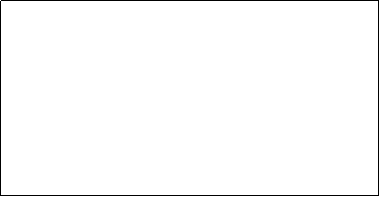Area of a Square              Area of a Rectangle              Area of a TriangleAREA of a RECTANGLE = the LENGTH  times the WIDTH. A = (l)(w) Find the area for the following rectangles: 1) A rectangle with a length of 6 m and a width of 3 m:     A=18m2       2) A rectangle with a length of 6 cm and a width of 4 cm:       A=24cm2       3) A rectangle with a length of 3x and a width of 2x:     A=6x2